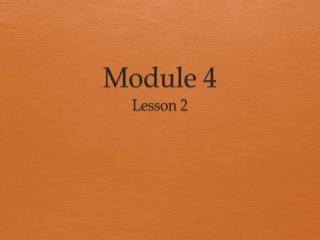DownloadDownload PresentationModule 4

# Module 4

Download Presentation## Module 4

- - - - - - - - - - - - - - - - - - - - - - - - - - - E N D - - - - - - - - - - - - - - - - - - - - - - - - - - -
##### Presentation Transcript

1. Module 4 Lesson 2

2. Objective • Add and subtract multiples of 10 including counting on to subtract.

3. Place Value Materials: Place value charts, personal white boards • Show 1 ten and 3 ones in number disks. Write the number below it. • Say the number in unit form. • 1 ten 3 ones. • Say the number in standard form. • 13.

4. Place Value Continued • Add 2 tens to your chart. How many tens do you have now? • What is 20 more than 13? • Add 3 tens to 33. How many tens do you have now? • 6 tens. • What is 30 more than 33? • Say the number in unit form. • 6 tens 3 ones. • Now subtract 4 tens from 63. What is 40 less than 63? • 23.

5. How many more tens? • If I say 34 ─ 24, you say 10. To say it in a sentence you say 34 is 10 more than 24. Ready? • 64 – 44. • Say it in a sentence. • 64 is 20 more than 44. 68 – 38 59 – 49 47 – 17 85 – 45 99 – 19

6. Concept Development • Yesterday we added and subtracted 1 ten. Today, let’s add 2 tens, then 3 tens, and more! • How many do you see? • The Say Ten way? • What would have if I were to add 2 more tens? Turn and talk. • What is 34 + 20? The say ten way.

7. Concept Development Continued The Arrow Way • If I asked you to add 3 tens to 26, how could you solve that? • Let’s show that on the board using both simplifying strategies, the arrow way and number bonds. I know many of you can just do mental math! • I can write adding 3 tens the arrow way, as we did yesterday. • I can also break apart the tens and ones with a number bond, add the tens and then add the ones. • No matter which way I write it, when I add tens to a number, the ones stay the same! 26 +10 36 +10 46 +10 56 26 56 +30 Number Bonds 26 + 30 = 56 20 + 30 = 56 50 + 6 = 56 20 6 Mental Math 26 + 30 = 56

8. Concept Development Continued • Now it’s your turn. On your personal white board, solve 18 + 20. Show your board when you have an answer. • Let’s try a few others: 25 + 50 38 + 40 40 + 27

9. Concept Development Continued • Ok, now subtract 3 tens from 56. Take a moment and work on your personal board to solve 56 – 30.

10. Concept Development Continued • We have an extra simplifying strategy when we are subtracting. We can count up from the part we know. • What is the whole? • 56. • What is the part we know? • 30. • How could we show the missing part with an addition problem? • 30 + ___ = 56.  ___ + 30 = 56. • We can use the arrow way, counting first by either tens or ones. Try it with a partner.

11. Story Problem Susan has 57 cents in her piggy bank. If she just put in 30 cents today, how much did she have yesterday? • Think of the part to whole relationship! • RDW! • SHARE!